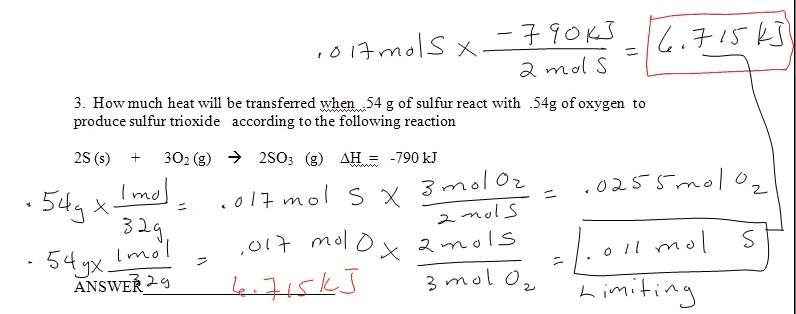# Thermochemistry Problem

## Homework Statement

How much heat will be transferred when 0.54g of sulfur reacts with 0.54g of oxygen to produce sulfur trioxide according to the following reaction:

2 S (s) + 3 O2 (g) --> 2 SO3 (g)

change in Enthalpy = -790kJ

## The Attempt at a Solution

First, I reduced the equation:

S + 3/2 O2 --> SO3
change in Enthalpy = -395 kJ/mol

Second, I figured out the limiting reactant:

0.54g x (mol/32.07g) = 0.017mol x (3 mol O2 / 2 mol S) = 0.0253 mol O2
0.54g O2 x (mol/32g) = 0.017mol x (2 mol S / 3 mol O2) = 0.0112 mol S

Third, I took the limiting reactant, 0.0112 mol S, and did:

0.0112 mol S x -395kJ = 4.42kJ

So my answer is 4.42kJ. However, this answer was incorrect. Would anyone be able to guide me to the correct answer? Thanks!!

Tom Mattson
Staff Emeritus
Gold Member
Second, I figured out the limiting reactant:

0.54g x (mol/32.07g) = 0.017mol x (3 mol O2 / 2 mol S) = 0.0253 mol O2
0.54g O2 x (mol/32g) = 0.017mol x (2 mol S / 3 mol O2) = 0.0112 mol S

When determining the limiting reactant, you are supposed to calculate the amount of product that results by the complete consumption of each reactant separately. That means that you shouldn't be doing stoichiometric calculations with S and O2. Rather, you should be doing calculations to determine how much SO3 each reactant can produce.

Third, I took the limiting reactant, 0.0112 mol S, and did:

According to my calculation, O2 is the limiting reactant.

Okay, so how does this look?

0.017 mol S ---- which produces 0.017 mol SO3
0.017 mol O2 ---- which produces 0.011 mol SO3

Therefore, the 0.017 mol O2 is the limiting reactant because using all 0.017 mol O2 only produces 0.011 mol SO3.

What exactly do I do next?

Thanks.

Tom Mattson
Staff Emeritus
Gold Member
You want to know the enthalphy change that results from using 0.54 g of O2, and you know the enthalpy change that results from using 3 mol of O2. Seems pretty straightforward, does it not?

I am probably making a silly mistake, but for some reason, I am still getting -4.47kJ as my answer.

0.017 mol O2 x ( -790kJ / 3 mol O2 ) = -4.47 kJ

Thanks again for the assistance, Tom Mattson.

Tom Mattson
Staff Emeritus
Gold Member
Are you submitting your answers in to some computer grading system? If so, then maybe it's just being picky. My calculation disagrees with yours in the hundredths place.

$$0.54gO_2\times\frac{1molO_2}{32gO_2}\times\frac{-790kJ}{3molO_2}=-4.44375kJ$$

This was a quiz in real life. The teacher provided us with the work and answer for each problem. I uploaded the work and answer for this particular problem in an image file:Tom Mattson
Staff Emeritus